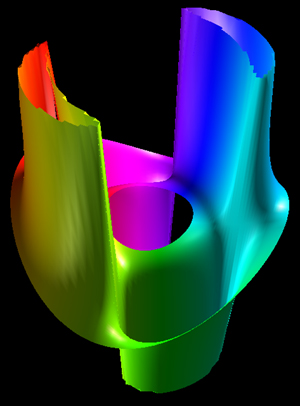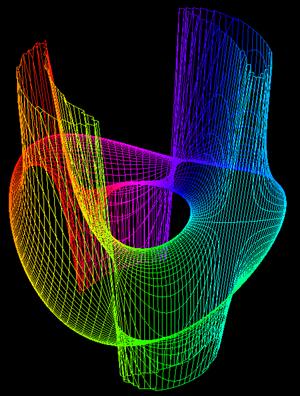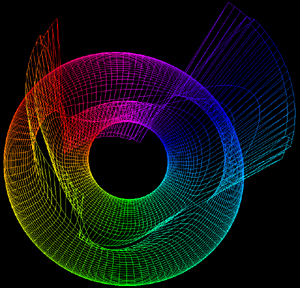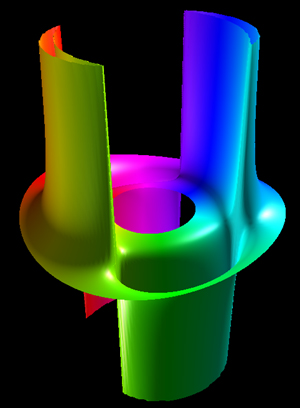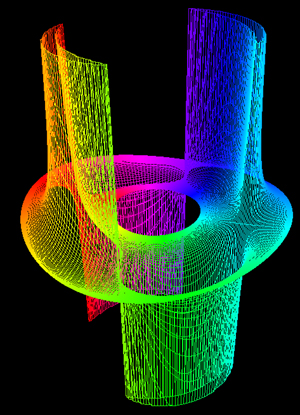# Pseudocatenoid

By Roger Bagula
Graphics by Paul Bourke
January 2003
x = cos(u) (2 + cos(v))
y = sin(u) (2 + cos(v))
z = x2 - y2 + 2 x y tan2(v)
-pi <= u <= pi, -pi <= v <= pi